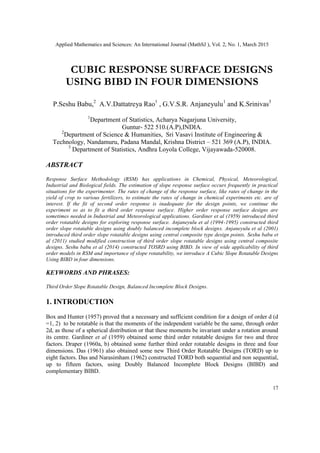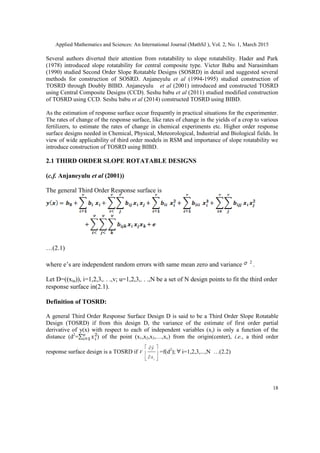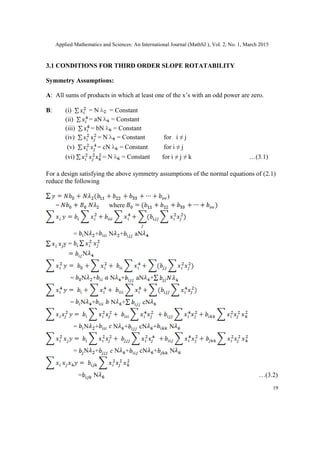Successfully reported this slideshow.

# Cubic Response Surface Designs Using Bibd in Four Dimensions×

# Cubic Response Surface Designs Using Bibd in Four Dimensions

Response Surface Methodology (RSM) has applications in Chemical, Physical, Meteorological, Industrial and Biological fields. The estimation of slope response surface occurs frequently in practical situations for the experimenter. The rates of change of the response surface, like rates of change in the yield of crop to various fertilizers, to estimate the rates of change in chemical experiments etc. are of
interest. If the fit of second order response is inadequate for the design points, we continue the
experiment so as to fit a third order response surface. Higher order response surface designs are sometimes needed in Industrial and Meteorological applications. Gardiner et al (1959) introduced third order rotatable designs for exploring response surface. Anjaneyulu et al (1994-1995) constructed third order slope rotatable designs using doubly balanced incomplete block designs. Anjaneyulu et al (2001)
introduced third order slope rotatable designs using central composite type design points. Seshu babu et al (2011) studied modified construction of third order slope rotatable designs using central composite
designs. Seshu babu et al (2014) constructed TOSRD using BIBD. In view of wide applicability of third
order models in RSM and importance of slope rotatability, we introduce A Cubic Slope Rotatable Designs Using BIBD in four dimensions.

Response Surface Methodology (RSM) has applications in Chemical, Physical, Meteorological, Industrial and Biological fields. The estimation of slope response surface occurs frequently in practical situations for the experimenter. The rates of change of the response surface, like rates of change in the yield of crop to various fertilizers, to estimate the rates of change in chemical experiments etc. are of
interest. If the fit of second order response is inadequate for the design points, we continue the
experiment so as to fit a third order response surface. Higher order response surface designs are sometimes needed in Industrial and Meteorological applications. Gardiner et al (1959) introduced third order rotatable designs for exploring response surface. Anjaneyulu et al (1994-1995) constructed third order slope rotatable designs using doubly balanced incomplete block designs. Anjaneyulu et al (2001)
introduced third order slope rotatable designs using central composite type design points. Seshu babu et al (2011) studied modified construction of third order slope rotatable designs using central composite
designs. Seshu babu et al (2014) constructed TOSRD using BIBD. In view of wide applicability of third
order models in RSM and importance of slope rotatability, we introduce A Cubic Slope Rotatable Designs Using BIBD in four dimensions.